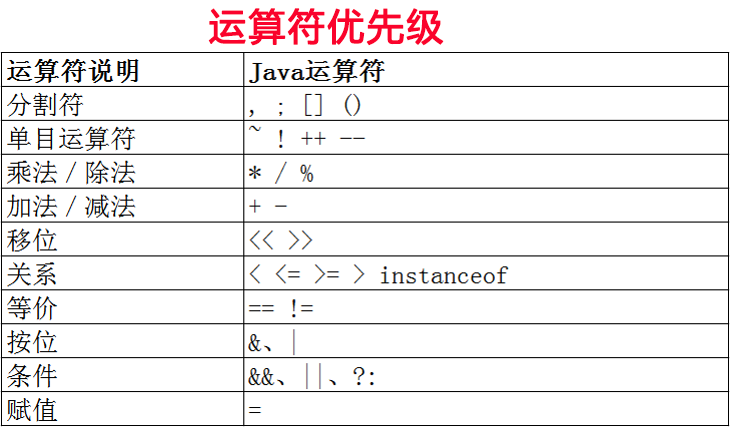运算符的优先级

一、优先级的规律

• 第一，遵循运算符优先级，也就是下图中 上面运算符 的比 下面运算符 的优先级高。

• 第二，从左向右，比如 a + b + c，先计算 a + b， 然后用结果再去 + c

• 通过 （） 可以改变优先级，比如 a + b * c 和 （a + b）* c 是不一样的，和我们小学数学类似

一、优先级介绍二、代码实战

package com.qicong.cj;

/**
* User: 祁大聪
*/
public class C19 {

public static void main(String[] args) {

int a = 10 , b = 90, c = 10, d = 2;

int sum = a + b + c + d; //112
System.out.println("sum = " + sum);

sum = a + b * c + d;
System.out.println("sum = " + sum);

sum = (a + b) * c + d;
System.out.println("sum = " + sum);

sum = a + b * ++c + d;
System.out.println("sum = " + sum);

if(a > b && (a < c || b < c) || c > a){
//todo
}

}
}

• 目录